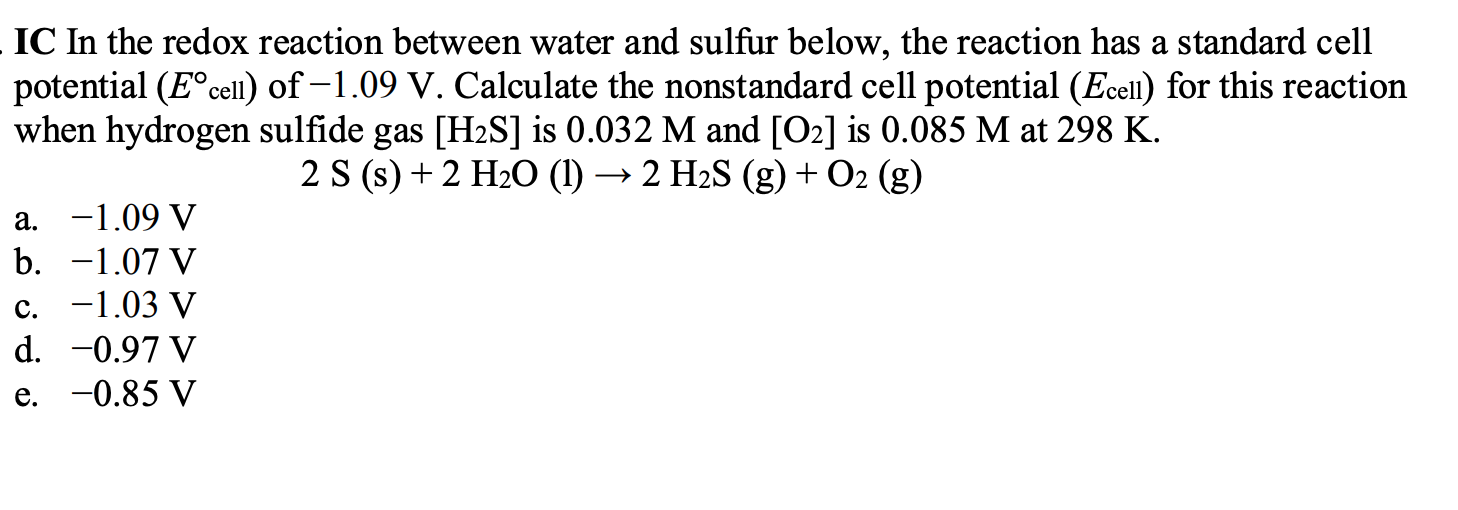# In the redox reaction between water and sulfur below, the reaction has a standard cell potential (Eocell) of -1.09 V. Calculate the nonstandard cell potential (Ecell) for this reaction when hydrogen sulfide gas [H2S] is 0.032 M and [O2] is 0.085 M at 298 K. 2 S(s) + 2 H2O(l) --> 2 H2S(g) + O2(g)